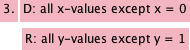### Home > AC > Chapter 11 > Lesson 11.1.5 > Problem11-54

11-54.

For each relation graphed in problem 11-53, name the domain and the range.

Domain = x-values Range = y-values

$\left. \begin{array} { l } { D : - \infty < x < \infty } \\ { R : = - \infty < y < \infty } \end{array} \right.$

$\left. \begin{array} { l } { D : - 4 \leq x \leq 0 } \\ { R : = 0 \leq y \leq 2 } \end{array} \right.$Try the rest on your own.